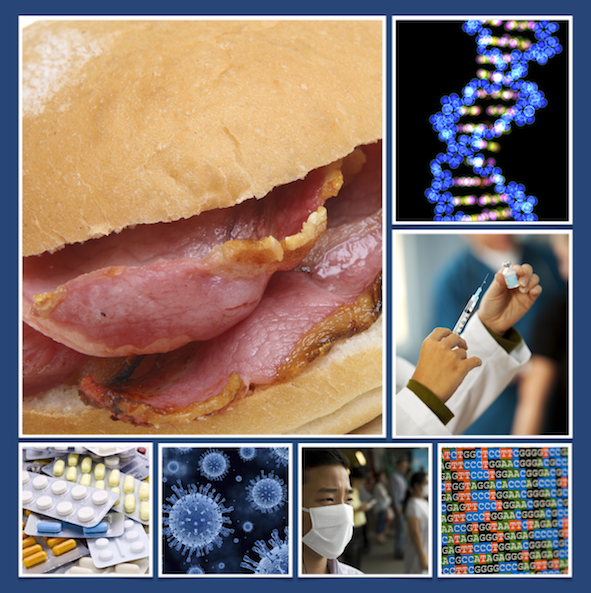# Maths and Our Health - Motivate Resource Packs

##### Age 11 to 16Biomedical science provides rich contexts for studying maths. The five resource packs linked below were originally produced in 2010 as part of a project funded by the Wellcome Trust. They are based around biomedical topics which support and extend the maths curriculum at secondary level (11-16 year-olds).  These packs show how maths underpins cutting edge biomedical research.  They also introduce students to important ethical issues.

Each pack consists of short video clips in which an expert talks about their work and the topic.  Additional resources - presentations, worksheets, games - help students to explore the topic in greater depth.  There are also teacher's notes and worksheet answers for each pack.

 Topic Curriculum Areas "Eating bacon sandwiches is bad for you!" Evaluating risk Percentage calculations Interpreting graphs Ratio and proportion Basic probability Epidemics: modelling with mathematics Maths in context (mathematical modelling) Interpreting graphs Life-saving maths: how does vaccination work? Probability Interpreting graphs Creating and testing a mathematical model The economics of health: how do we decide? Multi-step problems Using graphs and equations to solve problems Manipulating equations Probability and tree diagrams Strategic thinking The test is positive - but what are the odds it's wrong? Probability Independence and dependence Conditional probability using contingency tables and tree diagrams (and Venn diagrams)